Blaschke-Santaló inequality

One of the most important affine isoperimetric inequalities (cf. Isoperimetric inequality). It has applications to number theory, differential equations, differential geometry, stochastic geometry, as well as in the study of Banach spaces.

The Blaschke–Santaló inequality states that ifis a convex body (a compact convex subset with non-empty interior) in Euclidean-spacesuch that the centroid ofis at the origin, then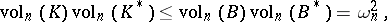with equality if and only ifis an ellipsoid. Here,denotes-dimensional volume,denotes the unit ball centred at the origin,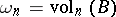, and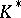denotes the polar body of: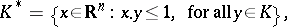where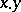denotes the standard inner product ofand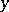in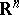.

The Blaschke–Santaló inequality was proven by W. Blaschke in 1917, for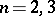, and by L. Santaló in 1949, for all. Both proofs use the affine isoperimetric inequality of affine differential geometry and the solution of the Minkowski problem. The fact that only ellipsoids attain the upper bound in the Blaschke–Santaló inequality was demonstrated by J. Saint Raymond in 1981 for the class of bodies symmetric about the origin and, in general, by C. Petty in 1985. In the 1980s, new and simpler proofs of the Blaschke–Santaló inequality were discovered by Saint Raymond, K. Ball, and M. Meyer and A. Pajor. (All facts can be found in, e.g., [a2] and [a3].)

In the plane (), the complementary inequality to the Blaschke–Santaló inequality was obtained by K. Mahler in 1939. Mahler's inequality is the special caseof the following conjectured inequality: Ifis a convex body in, then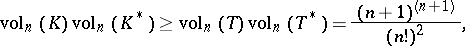whereis a simplex whose centroid is at the origin. For bodies, symmetric about the origin, the conjectured complementary inequality is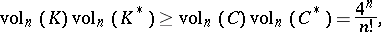where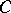is the cube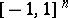. Again, forthis inequality was established by Mahler in 1939. Reisner's inequality is precisely this last inequality for the special case whenis a zonoid.

A major breakthrough occurred when J. Bourgain and V.D. Milman [a1] showed that this last conjectured inequality is, at least asymptotically, correct. By using techniques from the local theory of Banach spaces, they proved the existence of a constant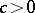, independent of, such that for each convex bodythat is symmetric about the origin: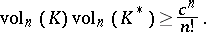Of the many applications of the Blaschke–Santaló inequality, one of its most immediate consequences provides an excellent upper bound for the volume of a convex body in terms of the length of the projections of the body onto lines. Consider the following conjectured inequality: Ifis a convex body in, thenwith equality if and only ifis an ellipsoid. Here,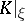denotes the (image of) the orthogonal projection ofonto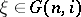, and the integration is over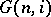, the Grassmann manifold (Grassmannian) of-dimensional subspaces of, with respect to its usual invariant probability measure. For the special case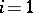this inequality is an easy consequence of the Blaschke–Santaló inequality. In fact, for bodies symmetric about the origin this inequality is the Blaschke–Santaló inequality! For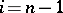this inequality was established in 1972 and is known as the Petty projection inequality. The complementary inequality to the Petty projection inequality was established by G. Zhang in 1991. All the cases where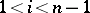are open.

In [a2] it is shown how the Blaschke–Santaló inequality, the curvature image inequality, Petty's geo-minimal surface area inequality, and the affine isoperimetric inequality of affine differential geometry, are very closely related in that given any one of these inequalities, then all the others can be easily obtained by well-known methods.